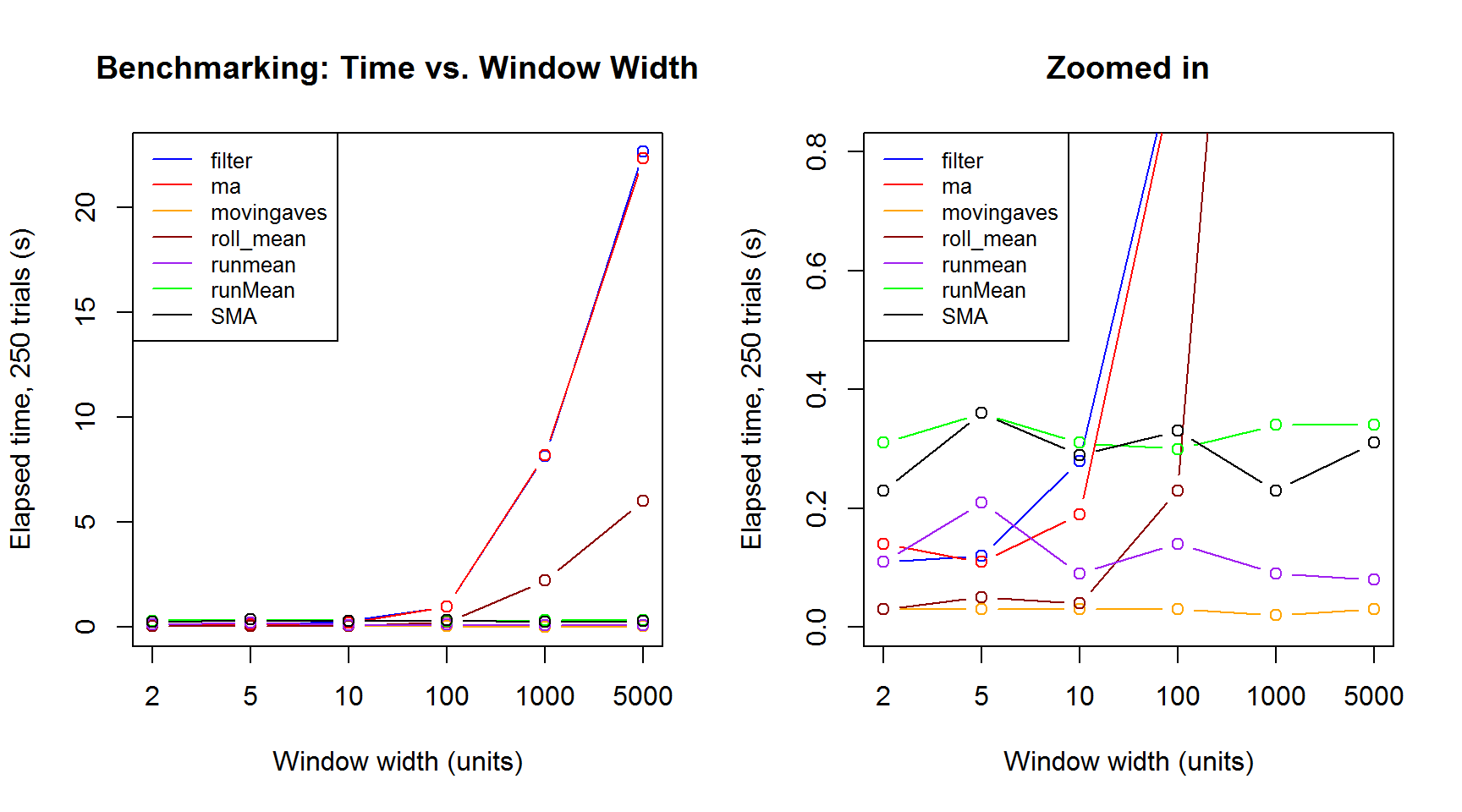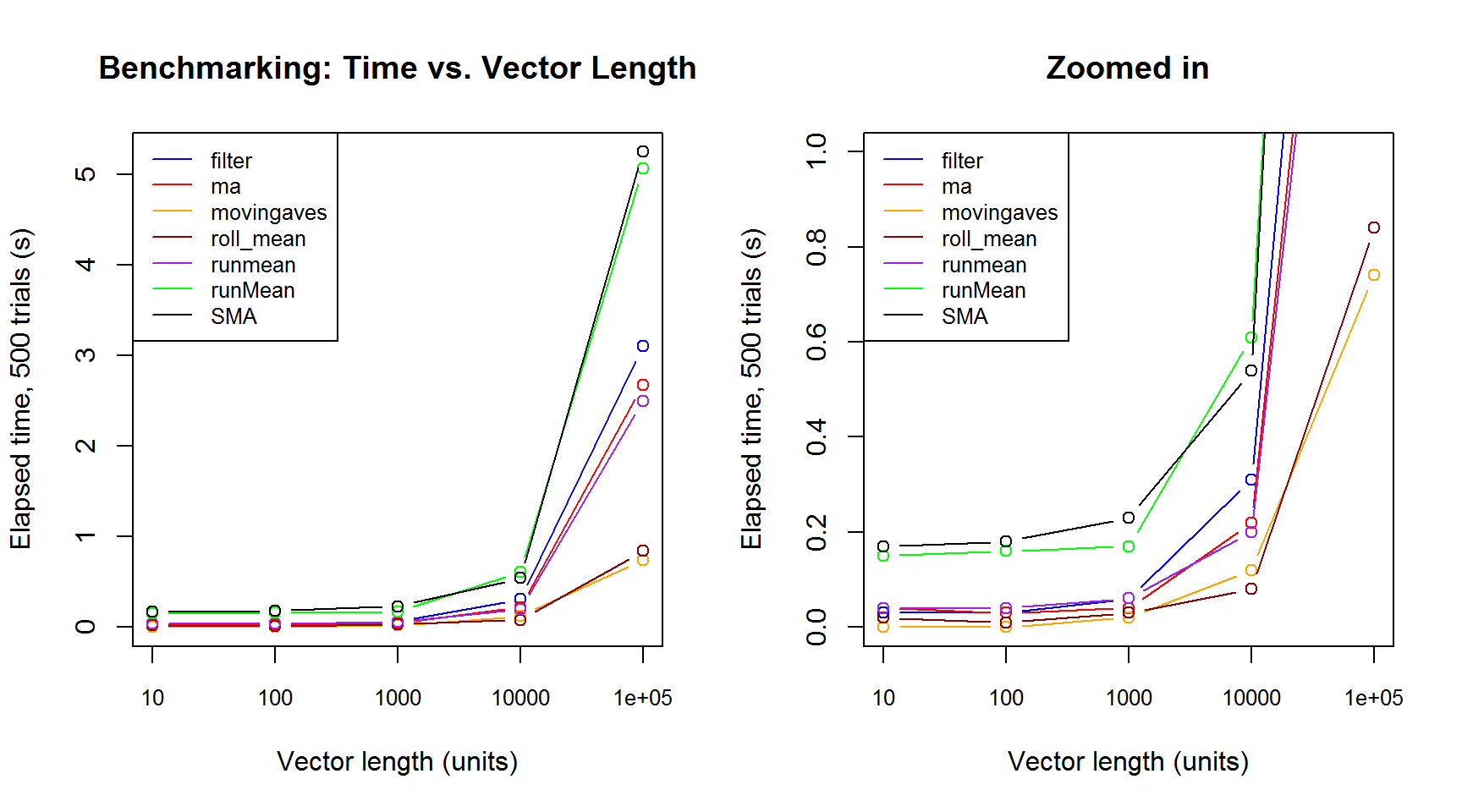There are quite a few R functions/packages for calculating moving averages. The purpose of this article is to compare a bunch of them and see which is fastest.

Here are the 10 functions I’ll be looking at, in alphabetical order (Disclaimer: the accelerometry package is mine).

• filter in package stats (part of R install)
• ma in package forecast
• movavg in package pracma
• movingaves in package accelerometry
• roll_mean in package RcppRoll
• rollapply in package zoo
• rollmean in package zoo
• runmean in package caTools
• runMean in package TTR
• SMA in package TTR

First, here is the syntax for each function:

# Sample 1,000 values from Poisson(3)
set.seed(1)
x <- rpois(n = 1000, lambda = 3)

# Calculate 5-unit moving average using each of the functions
width <- 5

y.filter <- filter(x = x, filter = rep(1, width)) / width
y.filter <- y.filter[! is.na(y.filter)]

library("forecast")
y.ma <- ma(x = x, order = width)
y.ma <- y.ma[! is.na(y.ma)]

library("pracma")
y.movavg <- movavg(x = x, n = width, type = "s")
y.movavg <- y.movavg[-c(1: (width - 1))]

library("accelerometry")
y.movingaves <- movingaves(x = x, window = width)

library("RcppRoll")
y.roll_mean <- roll_mean(x = x, n = width)

library("zoo")
y.rollapply <- rollapply(data = x, width = width, FUN = mean)
y.rollmean <- rollmean(x = x, k = width)

library("caTools")
y.runmean <- runmean(x = x, k = width, endrule = "trim")

library("TTR")
y.runMean <- runMean(x = x, n = width)
y.runMean <- y.runMean[! is.na(y.runMean)]

y.SMA <- SMA(x = x, n = width)
y.SMA <- y.SMA[! is.na(y.SMA)]

There were some minor differences among functions, i.e. whether they have extra NA’s in front or back, but I took care of that in the above code. All methods indeed give the same results:

# Combine all of the moving average vectors into a matrix
y.all <- cbind(y.filter, y.ma, y.movavg, y.movingaves, y.roll_mean,
y.rollapply, y.rollmean, y.runmean, y.runMean, y.SMA)

# Other than a small precision issue, all moving averages are the same
max(apply(y.all, 1, function(x) diff(range(x))))
##  8.881784e-16

Let’s see which gives the fastest performance over 500 trials:

library("rbenchmark")
(test1 <- benchmark(filter(x = x, filter = rep(1, width)) / width,
ma(x = x, order = width),
movavg(x = x, n = width, type = "s"),
movingaves(x = x, window = width),
roll_mean(x = x, n = width),
rollapply(data = x, width = width, FUN = mean),
rollmean(x = x, k = width),
runmean(x = x, k = width, endrule = "trim"),
runMean(x = x, n = width),
SMA(x = x, n = width),
replications = 500, order = "elapsed",
columns = c("test", "replications", "elapsed")))
##                                              test replications elapsed
## 4               movingaves(x = x, window = width)          500    0.02
## 5                     roll_mean(x = x, n = width)          500    0.02
## 2                        ma(x = x, order = width)          500    0.04
## 8     runmean(x = x, k = width, endrule = "trim")          500    0.05
## 1     filter(x = x, filter = rep(1, width))/width          500    0.06
## 9                       runMean(x = x, n = width)          500    0.20
## 10                          SMA(x = x, n = width)          500    0.20
## 7                      rollmean(x = x, k = width)          500    0.37
## 6  rollapply(data = x, width = width, FUN = mean)          500    0.56
## 3            movavg(x = x, n = width, type = "s")          500    3.53

I’ll do another quick test on a vector of non-integer values:

# Sample 1,000 values from N(0, 1)
set.seed(1)
x <- rnorm(n = 1000)

(test2 <- benchmark(filter(x = x, filter = rep(1, width)) / width,
ma(x = x, order = width),
movavg(x = x, n = width, type = "s"),
movingaves(x = x, window = width),
roll_mean(x = x, n = width),
rollapply(data = x, width = width, FUN = mean),
rollmean(x = x, k = width),
runmean(x = x, k = width, endrule = "trim"),
runMean(x = x, n = width),
SMA(x = x, n = width),
replications = 500, order = "elapsed",
columns = c("test", "replications", "elapsed")))
##                                              test replications elapsed
## 4               movingaves(x = x, window = width)          500    0.01
## 5                     roll_mean(x = x, n = width)          500    0.02
## 2                        ma(x = x, order = width)          500    0.04
## 1     filter(x = x, filter = rep(1, width))/width          500    0.05
## 8     runmean(x = x, k = width, endrule = "trim")          500    0.07
## 10                          SMA(x = x, n = width)          500    0.19
## 9                       runMean(x = x, n = width)          500    0.21
## 7                      rollmean(x = x, k = width)          500    0.42
## 6  rollapply(data = x, width = width, FUN = mean)          500    0.50
## 3            movavg(x = x, n = width, type = "s")          500    3.50

It looks like movavg, rollapply, and rollmean are slower than the others, so let’s disqualify those three and continue to another scenario: a longer vector and a longer window length.

# Sample 50,000 values from N(0, 1)
set.seed(1)
x <- rnorm(n = 50000)

width <- 500
(test3 <- benchmark(filter(x = x, filter = rep(1, width)) / width,
ma(x = x, order = width),
movingaves(x = x, window = width),
roll_mean(x = x, n = width),
runmean(x = x, k = width, endrule = "trim"),
runMean(x = x, n = width),
SMA(x = x, n = width),
replications = 500, order = "elapsed",
columns = c("test", "replications", "elapsed")))
##                                          test replications elapsed
## 3           movingaves(x = x, window = width)          500    0.22
## 5 runmean(x = x, k = width, endrule = "trim")          500    1.09
## 6                   runMean(x = x, n = width)          500    2.11
## 7                       SMA(x = x, n = width)          500    2.15
## 4                 roll_mean(x = x, n = width)          500   11.87
## 2                    ma(x = x, order = width)          500   45.40
## 1 filter(x = x, filter = rep(1, width))/width          500   45.71

Here movingaves looks like the clear winner. It’s interesting that roll_mean fell so far in this test. It seems to become progressively less efficient as the window size grows. Actually, let’s look directly at how window length affects speed for a fixed vector length.

# Look at effect of window size on speed of various functions
x <- rpois(n = 10000, lambda = 5)
width.vals <- c(2, 5, 10, 100, 1000, 5000)
benchmark.results <- matrix(NA, ncol = 7, nrow = length(width.vals))
for (ii in 1: length(width.vals)) {
width.ii <- width.vals[ii]
benchmark.results[ii, ] <-
benchmark(filter(x = x, filter = rep(1, width.ii)) / width.ii,
ma(x = x, order = width.ii),
movingaves(x = x, window = width.ii),
roll_mean(x = x, n = width.ii),
runmean(x = x, k = width.ii, endrule = "trim"),
runMean(x = x, n = width.ii),
SMA(x = x, n = width.ii),
replications = 250)$elapsed } # Create figure cols <- c("blue", "red", "orange", "darkred", "purple", "green", "black") par(mfrow = c(1, 2)) plot(benchmark.results[, 1], main = "Benchmarking: Time vs. Window Width", ylab = "Elapsed time, 250 trials (s)", xlab = "Window width (units)", ylim = c(0, max(benchmark.results)), type = "n", xaxt = "n") axis(side = 1, at = 1: 6, labels = width.vals) for (ii in 1: 7) { points(x = benchmark.results[, ii], type = "b", col = cols[ii]) } legend("topleft", lty = 1, col = cols, cex = 0.8, legend = c("filter", "ma", "movingaves", "roll_mean", "runmean", "runMean", "SMA")) # Create zoomed-in version plot(benchmark.results[, 1], main = "Zoomed in", ylab = "Elapsed time, 250 trials (s)", xlab = "Window width (units)", ylim = c(0, 0.8), type = "n", xaxt = "n") axis(side = 1, at = 1: 6, labels = width.vals) for (ii in 1: 7) { points(x = benchmark.results[, ii], type = "b", col = cols[ii]) } legend("topleft", lty = 1, col = cols, cex = 0.8, legend = c("filter", "ma", "movingaves", "roll_mean", "runmean", "runMean", "SMA"))Note that the x-axis is not to scale; the results were easier to visualize this way. Overall, movingaves and roll_mean are similarly fast for relatively small window widths, but movingaves is easily the fastest when the window width gets larger. For one last analysis, let’s see how the length of the vector affects the results, holding the window width fixed at 5 units. I’ll move back to 500 trials for these simulations, as they’re somewhat faster. # Look at effect of vector length on speed of various functions length.vals <- c(10, 100, 1000, 10000, 100000) width <- 5 benchmark.results <- matrix(NA, ncol = 7, nrow = length(length.vals)) for (ii in 1: length(length.vals)) { length.ii <- length.vals[ii] x <- rpois(n = length.ii, lambda = 5) benchmark.results[ii, ] <- benchmark(filter(x = x, filter = rep(1, width)) / width, ma(x = x, order = width), movingaves(x = x, window = width), roll_mean(x = x, n = width), runmean(x = x, k = width, endrule = "trim"), runMean(x = x, n = width), SMA(x = x, n = width), replications = 500)$elapsed
}

# Create figure
cols <- c("blue", "red", "orange", "darkred", "purple", "green", "black")
par(mfrow = c(1, 2))
plot(benchmark.results[, 1],
main = "Benchmarking: Time vs. Vector Length",
ylab = "Elapsed time, 500 trials (s)",
xlab = "Vector length (units)",
ylim = c(0, max(benchmark.results)),
type = "n",
xaxt = "n")
axis(side = 1, at = 1: 5, labels = length.vals, cex.axis = 0.8)
for (ii in 1: 7) {
points(x = benchmark.results[, ii], type = "b", col = cols[ii])
}
legend("topleft", lty = 1, col = cols, cex = 0.8,
legend = c("filter", "ma", "movingaves", "roll_mean", "runmean",
"runMean", "SMA"))

# Create zoomed-in version
plot(benchmark.results[, 1],
main = "Zoomed in",
ylab = "Elapsed time, 500 trials (s)",
xlab = "Vector length (units)",
ylim = c(0, 1),
type = "n",
xaxt = "n")
axis(side = 1, at = 1: 5, labels = length.vals, cex.axis = 0.8)
for (ii in 1: 7) {
points(x = benchmark.results[, ii], type = "b", col = cols[ii])
}
legend("topleft", lty = 1, col = cols, cex = 0.8,
legend = c("filter", "ma", "movingaves", "roll_mean", "runmean",
"runMean", "SMA"))Here, movingaves and roll_mean are again the two fastest, with movingaves achieving a small speed advantage as the vector length gets larger. It’s interesting to see that the other 5 functions really drop off in performance for large vectors.

In summary, surprise surprise, I’m going to recommend movingaves in my accelerometry package for calculating moving averages efficiently in R.